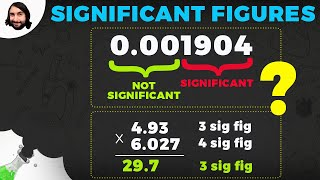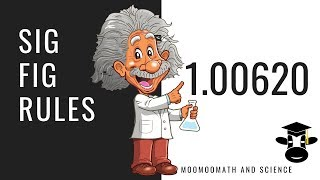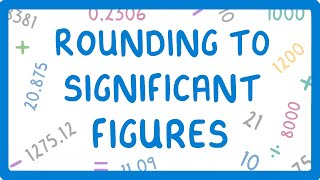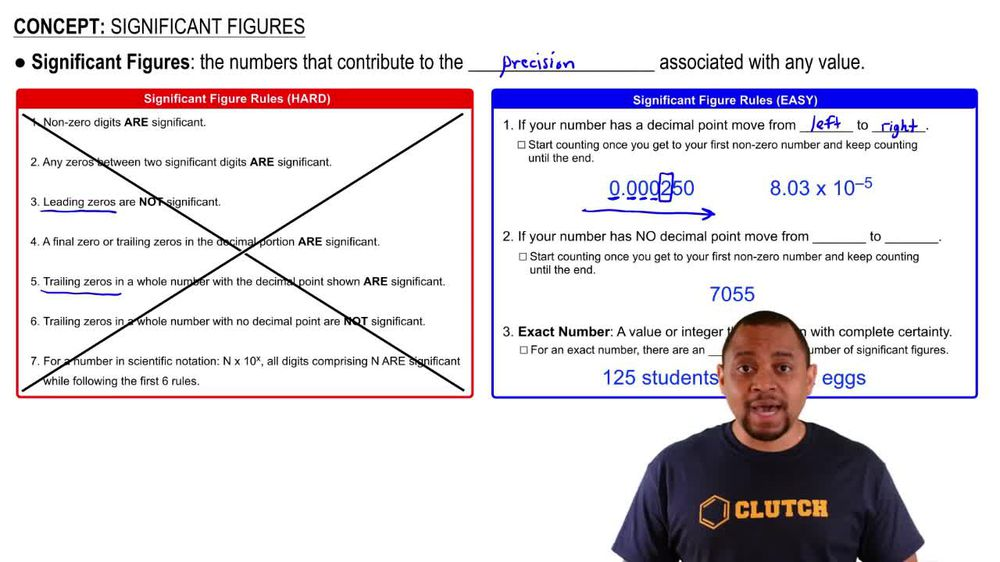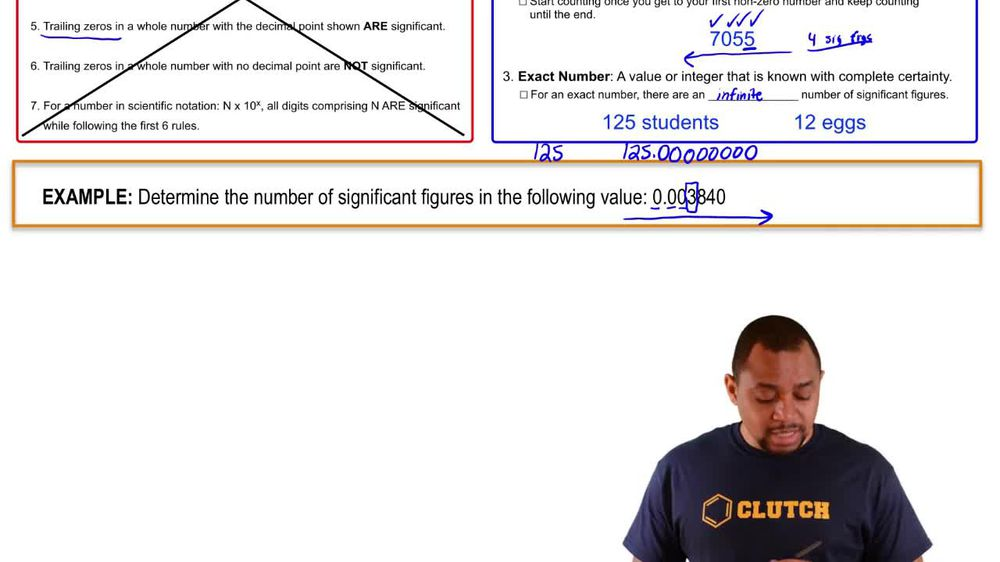Start typing, then use the up and down arrows to select an option from the list.
1. 1. Intro to General Chemistry2. Significant Figures
Problem

# For each number, underline the zeroes that are significant and draw an x through the zeroes that are not. a. 1,050,501 km b. 0.0020 m c. 0.000000000000002 s d. 0.001090 cm

Relevant Solution47s
Play a video:
Alright, so in this practice problem we're giving this number right here and we want to know how many significant figures does it have? All right, so here this number has a decimal point. So we are going to start counting for the significant numbers from left to the right. Okay. And we're going to start counting with the first non zero number, which is eight. Right? So from eight on all of these right here are going to be significant. Now, whenever we have a number with in scientific notation, the times 10, obviously it does not count as the number of significant figures. So here we have 123456, right trailing zeros are significant. So we do have six uh significant figures in this number. Right, folks, thank you so much for watching.